# AP SSC 10th Class Maths Solutions Chapter 13 Probability Ex 13.2

AP State Board Syllabus AP SSC 10th Class Maths Textbook Solutions Chapter 13 Probability Ex 13.2 Textbook Questions and Answers.

## AP State Syllabus SSC 10th Class Maths Solutions 13th Lesson Probability Exercise 13.2

### 10th Class Maths 13th Lesson Probability Ex 13.2 Textbook Questions and Answers

Question 1.
A bag contains 3 red balls and 5 black balls. A ball is drawn at random from the bag. What is the probability that the ball drawn is (i) red ? (ii) not red?
i) Total number of balls in the bag = 3 red + 5 black = 8 balls.
Number of total outcomes when a ball is drawn at random = 3 + 5 = 8
Now, number of favourable outcomes of red ball = 3.
∴ Probability of getting a red ball = $$\frac{\text { No. of favourable outcomes }}{\text { No. of total outcomes }}$$ = $$\frac{3}{8}$$
ii) If P( E) is the probability of drawing no red ball, then P(E) + P($$\overline{\mathrm{E}}$$) = 1
P($$\overline{\mathrm{E}}$$) = 1 – P(E)= 1 – $$\frac{3}{8}$$ = $$\frac{5}{8}$$Question 2.
A box contains 5 red marbles, 8 white marbles and 4 green marbles. One marble is taken out of the box at random. What is the probability that the marble taken out will be (i) red? (ii) white? (iii) not green?
Total number of marbles in the box = 5 red + 8 white + 4 green = 5 + 8 + 4= 17
Number of total outcomes in drawing a marble at random from the box =17.
i) Number of red marbles = 5
Number of favourable outcomes in drawing a red ball = 5
∴ Probability of getting a red ball P(R) = $$\frac{\text { No. of favourable outcomes }}{\text { No. of total outcomes }}$$
P(R) = $$\frac{5}{17}$$

ii) Number of white marbles = 8
Number of favourable outcomes in drawing a white marble = 8
∴ Probability of getting a white marble
P(W) = $$\frac{8}{17}$$

iii) Number of ‘non-green’ marbles = 5 red + 8 white = 5 + 8 = 13
Number of outcomes favourable to drawing a non-green marble =13.
∴ Probability of getting a non- green marble
P(non – green) = $$\frac{\text { No. of favourable outcomes }}{\text { No. of total outcomes }}$$
P(non – green) = $$\frac{13}{17}$$
Probability of getting a green ball = $$\frac{\text { No. of green balls }}{\text { Total no. of balls }}$$ = $$\frac{4}{17}$$
Now P(G) = $$\frac{4}{17}$$
and P(G) + P($$\overline{\mathrm{ G}}$$) = 1
∴ P($$\overline{\mathrm{G}}$$) = 1 – P(G)
= 1 – $$\frac{4}{17}$$
= $$\frac{13}{17}$$Question 3.
A Kiddy bank contains hundred 50p coins, fifty Rs. 1 coins, twenty Rs. 2 coins and ten Rs. 5 coins. If it is equally likely that one of the coins will fall out when the bank is turned upside down, what is the probability that the coin (i) will be a 50 p coin? (ii) will not be a Rs. 5 coin?
i) Number of 50 p coins = 100
Number of Rs. 1 coins = 50
Number of Rs. 2 coins = 20
Number of Rs. 5 coins = 10
Total number of coins = 180
Number of total outcomes for a coin to fall down = 180.
Number of outcomes favourable to 50 p coins to fall down = 100.
∴ Probability of a 50 p coin to fall down = $$\frac{\text { No. of favourable outcomes }}{\text { No. of total outcomes }}$$
= $$\frac{100}{180}$$ = $$\frac{5}{9}$$

ii) Let P(E) be the probability for a Rs. 5 coin to fall down.
Number of outcomes favourable to Rs. 5 coin = 10.
∴ Probability for a Rs. 5 coin to fall down = $$\frac{\text { No. of favourable outcomes }}{\text { No. of total outcomes }}$$
= $$\frac{10}{180}$$ = $$\frac{1}{18}$$
Then P($$\overline{\mathrm{E}}$$) is the probability of a coin which fall down is not a Rs. 5 coin.
Again P(E) + P($$\overline{\mathrm{E}}$$) = 1
∴ P($$\overline{\mathrm{E}}$$)= l-P(E)
= 1 – $$\frac{1}{18}$$
= $$\frac{17}{18}$$.Question 4.
Gopi buys a fish from a shop for his aquarium. The shopkeeper takes out one fish at random from a tank containing 5 male fish and 8 female fish (See figure). What is the probability that the fish taken out is a male fish?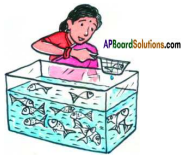Answer:
Number of male fish = 5
Number of female fish = 8
Total number of fish = 5 m + 8 f
= 13 fishes.
∴ Number of total outcomes in taking a fish at random from the aquarium =13.
Number of male fish = 5
∴ Number of outcomes favourable to male fish = 5.
∴ The probability of taking a male fish = $$\frac{\text { No. of favourable outcomes }}{\text { No. of total outcomes }}$$
= $$\frac{5}{13}$$
= 0.38

Question 5.
A game of chance consists of spinning an arrow which comes to rest pointing at one of the numbers 1, 2, 3, 4, 5, 6, 7, 8 (See figure), and these are equally likely outcomes. What is the probability that it will point at
(i) 8?
(ii) an odd number?
(iii) a number greater than 2?
(iv) a number less than 9?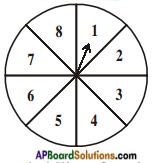Number of total outcomes are (1,2,……….., 8) = 8

i) Number of outcomes favourable to 8 = 1.
∴ P(8) = $$\frac{\text { No. of favourable outcomes }}{\text { No. of total outcomes }}$$
= $$\frac{1}{8}$$

ii) Number of ‘odd numbers’ on the spinning wheel = (1, 3, 5, 7) = 4
∴ Number of outcomes favourable to an odd number.
∴ Probability of getting an odd number = $$\frac{\text { No. of favourable outcomes }}{\text { No. of total outcomes }}$$
= $$\frac{4}{8}$$ = $$\frac{1}{2}$$

iii) Number greater than 2 are (3, 4, 5, 6, 7, 8)
Number of outcomes favourable to ‘greater than 2’ are = 6.
Probability of pointing a number greater than 2
P(E) = $$\frac{\text { No. of favourable outcomes }}{\text { No. of total outcomes }}$$
= $$\frac{6}{8}$$ = $$\frac{3}{4}$$

iv) Number less than 9 are: (1,2, 3, 4, 5, 6, 7, 8 …… 8)
∴ Number of outcomes favourable to pointing a number less than 9 = 8.
∴ Probability of a number less than 9
P(E) = $$\frac{\text { No. of outcomes favourable to less than } 9}{\text { No.of total outcomes }}$$
= $$\frac{8}{8}$$ = 1
Note : This is a sure event and hence probability is 1.Question 6.
One card is drawn from, a well-shuffled deck of 52 cards. Find the probability of getting
(i) a king of red colour
(ii) a face card
(iii) a red face card
(iv) the jack of hearts
(vi) the queen of diamonds.
Total number of cards = 52.
∴ Number of all possible outcomes in drawing a card at random = 52.
i) Number of outcomes favourable to the king of red colour = 2(♥ K, ♦ K)
∴ Probability of getting the king of red colour
P(E) = $$\frac{\text { No. of favourable outcomes }}{\text { No. of total outcomes }}$$
= $$\frac{2}{52}$$ = $$\frac{1}{26}$$

ii) Number of face cards in a deck of cards = 4 × 3 = 12 (K, Q, J)
Number of outcomes favourable to select a face card = 12.
∴ Probability of getting a face card
= $$\frac{\text { No. of favourable outcomes }}{\text { No. of total outcomes }}$$
= $$\frac{12}{52}$$ = $$\frac{3}{13}$$

iii) Number of red face cards = 2 × 3 = 6.
∴ Number of outcomes favourable to select a red face card = 6.
∴ Probability of getting a red face
= $$\frac{\text { No. of favourable outcomes }}{\text { No. of total outcomes }}$$
= $$\frac{6}{52}$$ = $$\frac{3}{26}$$

iv) Number of outcomes favourable to the jack of hearts = 1.
∴ Probability of getting jack of hearts
= $$\frac{\text { No. of favourable outcomes }}{\text { No. of total outcomes }}$$
= $$\frac{1}{52}$$

v) Number of spade cards = 13
∴ Number of outcomes favourable to ‘a spade card’ = 13.
∴ Probability of drawing a spade
= $$\frac{\text { No. of favourable outcomes }}{\text { No. of total outcomes }}$$
= $$\frac{13}{52}$$ = $$\frac{1}{4}$$

vi) Number of outcomes favourable to the queen of diamonds = 1.
∴ Probability of drawing the queen of diamonds
= $$\frac{\text { No. of favourable outcomes }}{\text { No. of total outcomes }}$$
= $$\frac{1}{52}$$Question 7.
Five cards-the ten, jack, queen, king and ace of diamonds, are well-shuffled with their face downwards. One card is then picked up at random.
i) What is the probability that the card is the queen?
ii) If the queen is drawn and put aside, what is the probability that the second card picked is (a) an ace? (b) a queen?
Total number of cards = 5.
∴ Number of total outcomes in picking up a card at random = 5.
i) Number of outcomes favourable to queen = 1.
∴ Probability of getting the queen
= $$\frac{\text { No.of outcomes favourable to the ‘Q’ }}{\text { No.of total outcomes }}$$
= $$\frac{1}{5}$$

ii) When queen is drawn and put aside, remaining cards are four.
∴ Number of total outcomes in drawing a card at random = 4.
a) Number of favourable outcomes to ace 1
Probability of getting an ace
= $$\frac{\text { No. of favourable outcomes }}{\text { No. of total outcomes }}$$
= $$\frac{1}{4}$$

b) Number of favourable outcomes to ‘Q’ = 0 (as it was already drawn and put aside)
∴ Probability that the card is Q = $$\frac{0}{4}$$ = 0
After putting queen aside, selecting the queen from the rest is an impossible event and hence the probability is zero.

Question 8.
12 defective pens are accidentally mixed with 132 good ones. It is not possible to just look at a pen and tell whether or not it is defective. One pen is taken out at random from this lot. Determine the probability that the pen taken out is a good one.
Number of good pens = 132
Number of defective pens = 12
Total number of pens = 132 + 12 = 144
∴ Total number of outcomes in taking a pen at random = 144.
No. of favourable outcomes in taking a good pen = 132.
∴ Probability of taking a good pen
= $$\frac{\text { No. of favourable outcomes }}{\text { No. of total outcomes }}$$
= $$\frac{132}{144}$$ = $$\frac{11}{12}$$Question 9.
A lot of 20 bulbs contain 4 defective ones. One bulb is drawn at random from the lot. What is the probability that this bulb is defective? Suppose the bulb drawn in previous case is not defective and is not replaced. Now one bulb is drawn at random from the rest. What is the probability that this bulb is not defective?
Given :
4 out of 20 bulbs are defective
(i.e.) Number of defective bulbs = 4
Number of non-defective bulbs = 20 – 4 = 16
If a bulb is drawn at random, the total outcomes are = 20
Number of outcomes favourable to ‘defective bulb’ = 4
∴ Probability of getting a defective bulb
= $$\frac{\text { No. of favourable outcomes }}{\text { No. of total outcomes }}$$
= $$\frac{4}{20}$$ = $$\frac{1}{5}$$
Suppose a non-defective bulb is drawn and not replaced, then the bulbs remaining are = 19
∴ Total outcomes in drawing a bulb from the rest = 19
Number of favourable outcomes in drawing non-defective bulb from the rest = 16 – 1 = 15
∴ Probability of getting a non-defective bulb in the second draw
= $$\frac{\text { No. of favourable outcomes }}{\text { No. of total outcomes }}$$
= $$\frac{15}{19}$$

Question 10.
A box contains 90 discs which are numbered from 1 to 90. If one disc is drawn at random from the box, find the probability that it bears
(i) a two digit number
(ii) a perfect square number
(iii) a number divisible by 5.
Total number of discs in the box = 90
∴ Number of total outcomes in drawing a disc at random from the box = 90.

i) Number of 2-digit numbers in the box (10, 11,….., 90) = 81
i.e., Number of favourable outcomes in drawing a 2 – digit numbers = 81
∴ Probability of selecting a disc bearing a 2 – digit number
= $$\frac{\text { No. of favourable outcomes }}{\text { No. of total outcomes }}$$
= $$\frac{81}{90}$$ = $$\frac{9}{10}$$ = 0.9

ii) Number of perfect squares in the box (12 = 1, 22 = 4, 32 = 9, 42 = 16, 52 = 25, 62 = 36, 72 = 49, 82 = 64 and 92 = 81) = 9
i.e., Number of favourable out-comes in drawning a disc bearing a perfect square = 9
∴ Probability of drawning a disc with a perfect square
= $$\frac{\text { No. of favourable outcomes }}{\text { No. of total outcomes }}$$
= $$\frac{9}{90}$$ = $$\frac{1}{10}$$

iii) Number of multiples of 5 from 1 to 90 are (5, 10, 15, ……….., 90) = 18
i.e., Number of favourable outcomes in drawing a disc with a multiple of 5 = 18
∴ Probability of drawing a disc bearing a number divisible by 5
= $$\frac{\text { No. of favourable outcomes }}{\text { No. of total outcomes }}$$
= $$\frac{18}{90}$$ = $$\frac{1}{5}$$Question 11.
Suppose you drop a die at random on the rectangular region shown in figure. What is the probability that it will land inside the circle with diameter 1 m?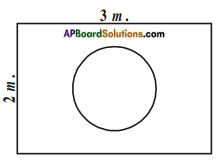3 m.
Length of the given rectangle = 3 m.
and its breadth = 2 m.
Area of the rectangle
= length × breadth = 3 × 2 = 6 m2
∴ Total area of the region for landing = 6 m2.
Diameter of the given circle = 1 m.
Area of the circle = $$\frac{\pi \mathrm{d}^{2}}{4}$$
= $$\frac{22}{7} \times \frac{1 \times 1}{4}\left[\text { or } \pi r^{2}=\frac{22}{7} \times \frac{1}{2} \times \frac{1}{2}\right]$$
= $$\frac{22}{28}$$
∴ Probability of the coin to land on the circle
= $$\frac{\frac{22}{28}}{6}$$
= $$\frac{22}{28×6}$$
= $$\frac{11}{28×3}$$
= $$\frac{11}{84}$$

Question 12.
A lot consists of 144 ball pens of which 20 are defective and the others are good. The shopkeeper draws one pen at random and gives it to Sudha. What is the probability that (i) She will buy it? (ii) She will not buy it?
Given : 20 out of 144 are defective i.e., no. of defective ball pens = 20
no. of good ball pens = 144 – 20 = 124
∴ Total outcomes in drawing a ball pen at random = 144.

i) Sudha buys it if it is not defective / a good one.
No. of outcomes favourable to a good pen = 124.
= $$\frac{\text { No. of favourable outcomes }}{\text { No. of total outcomes }}$$
= $$\frac{124}{144}$$ = $$\frac{31}{36}$$

ii) Sudha will not buy it-if it is a defective pen
No. of outcomes favourable to a defective pen = 20
∴ Probability of not buying it
= $$\frac{\text { No. of favourable outcomes }}{\text { No. of total outcomes }}$$
= $$\frac{20}{144}$$ = $$\frac{5}{36}$$

= 1 – $$\frac{31}{36}$$ = $$\frac{5}{36}$$Question 13.
Two dice are rolled simultaneously and counts are added
(i) Complete the table given below: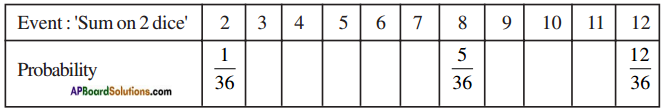(ii) A student argues that ‘there are 11 possible outcomes 2, 3, 4, 5, 6, 7, 8, 9, 10, 11 and 12. Therefore, each of them has a probability $$\frac{1}{11}$$. Do you agree with this argument? Justify your answer.
When two dice are rolled, total number of outcomes = 36 (see the given table).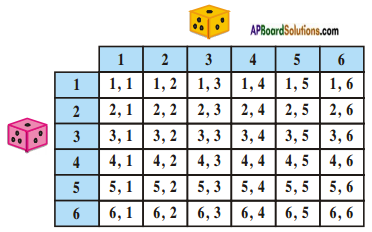(i)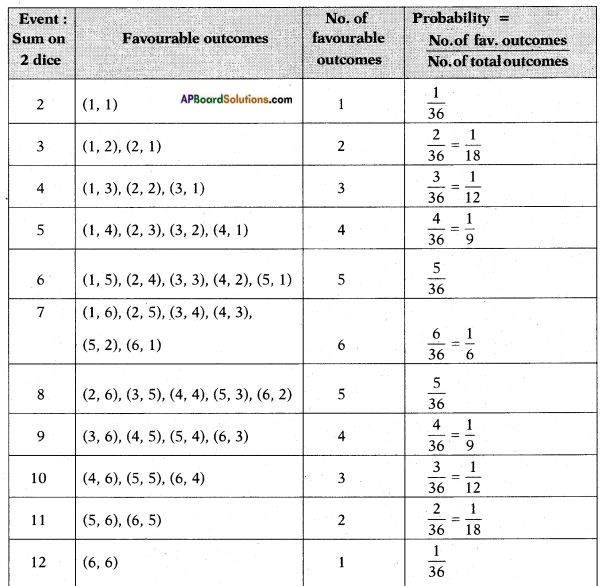(ii) The above (given) argument is wrong [from the above table].
The sum 2, 3, 4, ………… and 12 have different no. of favourable outcomes, moreover total number of outcomes are 36.Question 14.
A game consists of tossing a one rupee coin 3 times and noting its outcome each time. Hanif wins if all the tosses give the same result i.e., three heads or three tails, and loses otherwise. Calculate the probability that Hanif will lose the game.
When a coin is tossed for n – times, the total number of outcomes = 2n.
∴ If a coin is tossed for 3 – times, then the total number of outcomes = 23 = 8
Note the following :
TTT
TTH
THT
HTT
HHT
HTH
THH
HHH
Of the above, no. of outcomes with different results = 6.
Probability of losing the game
= $$\frac{\text { No. of favourable outcomes to lose }}{\text { No. of total outcomes }}$$
= $$\frac{6}{8}$$ = $$\frac{3}{4}$$

Question 15.
A dice is thrown twice. What is the probability that (i) 5 will not come up either time? (ii) 5 will come up atleast once? [Hint : Throwing a dice twice and throwing two dice simultaneously are treated as the same experiment].
If a dice is thrown n-times or n-dice are thrown simultaneously then the total
number of outcomes = 6×6×6….×6
(n – times) = 6n.
No. of total outcomes in throwing a dice for two times = 62 = 36.i) Let E be the event that 5 will not come up either time, then the favourable outcomes are
(1, 1), (1, 2), (1, 3), (1, 4), (1, 6), (2, 1), (2, 2), (2, 3), (2, 4), (2, 6), (3, 1), (3/2), (3, 3), (3, 4), (3, 6), (4, 1), (4, 2), (4, 3), (4, 4), (4, 6), (6, 1), (6, 2), (6, 3), (6, 4), (6, 6) = 25.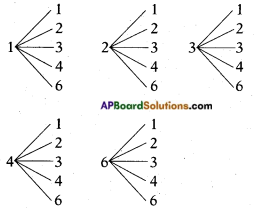∴ P(E) = $$\frac{\text { No. of favourable outcomes }}{\text { No. of total outcomes }}$$
= $$\frac{25}{36}$$ii) Let E be the event that 5 will come up atleast once.
Then the favourable outcomes are (1,5), (2, 5), (3, 5), (4, 5), (5, 5), (6, 5), (5, 1), (5, 2), (5, 3), (5, 4), (5, 6) = 11 No. of favourable outcomes = 11
∴ P(E) = $$\frac{\text { No. of favourable outcomes }}{\text { No. of total outcomes }}$$
= $$\frac{11}{36}$$